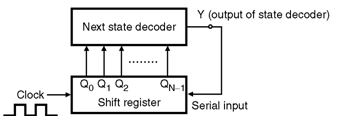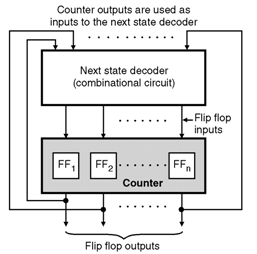Home > finite state machines > FSM Applications > Sequence Generator

# Sequence Generator

A sequence generator is a sequential circuit which generates a prescribed sequence at its output. The output sequence is in synchronication with the clock input. It is possible to design a sequence generator using counters or using the shift registers. Sequence Generator using Shift Register : The sequence generator is a circuit which generates a desired sequence bf bits at its output in synchronization with the clock. Some of the applications of the sequence generator are as follows :

1. Random bit generator
2. Counters
3. Code generators
4. Period and sequence generator.

Figure shows the basic structure of a sequence generator.The outputs of an N bit shift register (Q0 through QNâ€“1) are applied as inputs to a combinational circuit called â€œNext state decoderâ€. And the output (Y) of the next state decoder is applied to the serial input of the shift register. The â€œNext state decoderâ€ is designed according to the required sequence. Sequence Generator using Counters : â€¢ The general block diagram of a sequence generator using counter is shown in Figure below.The next state decoder is a combinational circuit. The input to it are obtained from the flip-flop outputs and its outputs are applied to the inputs of the flip-flops. Deciding the number of flip-flops : We will be given the required sequence of 1's and 0's that is to be generated such as 1011011 â€¦.

```Based on the given sequence we have to decide the number of flip-flops as follows.
1.	Count the number of 1's and 0's in the given sequence.
2.	Choose the higher number of the two. Let this number be N.
3.	The number of FFs (n) is then calculated as,
N  ï‚£   2n â€“ 1
```
Let us apply this to the given sequence, i.e. 1011011 â€¦ Number of 1's = 5, number of 0's = 2. So select higher one of them i.e. 5. So N = 5. But N = 2n â€“ 1 Hence 5 ï‚£ 2n â€“ 1 ïœ n = 4 â€¦ so four FFs will be required.